How to Set Axis Range (xlim, ylim) in Matplotlib - Stack Abuse

# How to Set Axis Range (xlim, ylim) in Matplotlib### Introduction

Matplotlib is one of the most widely used data visualization libraries in Python. Much of Matplotlib's popularity comes from its customization options - you can tweak just about any element from its hierarchy of objects.

In this tutorial, we'll take a look at how to set the axis range (xlim, ylim) in Matplotlib, to truncate or expand the view to specific limits.

### Creating a Plot

Let's first create a simple plot:

import matplotlib.pyplot as plt
import numpy as np

fig, ax = plt.subplots(figsize=(12, 6))

x = np.arange(0, 10, 0.1)
y = np.sin(x)
z = np.cos(x)

ax.plot(y, color='blue', label='Sine wave')
ax.plot(z, color='black', label='Cosine wave')

plt.show()


Here, we've plotted two sine functions, starting at 0 and ending at 100 with a step of 0.1. Running this code yields: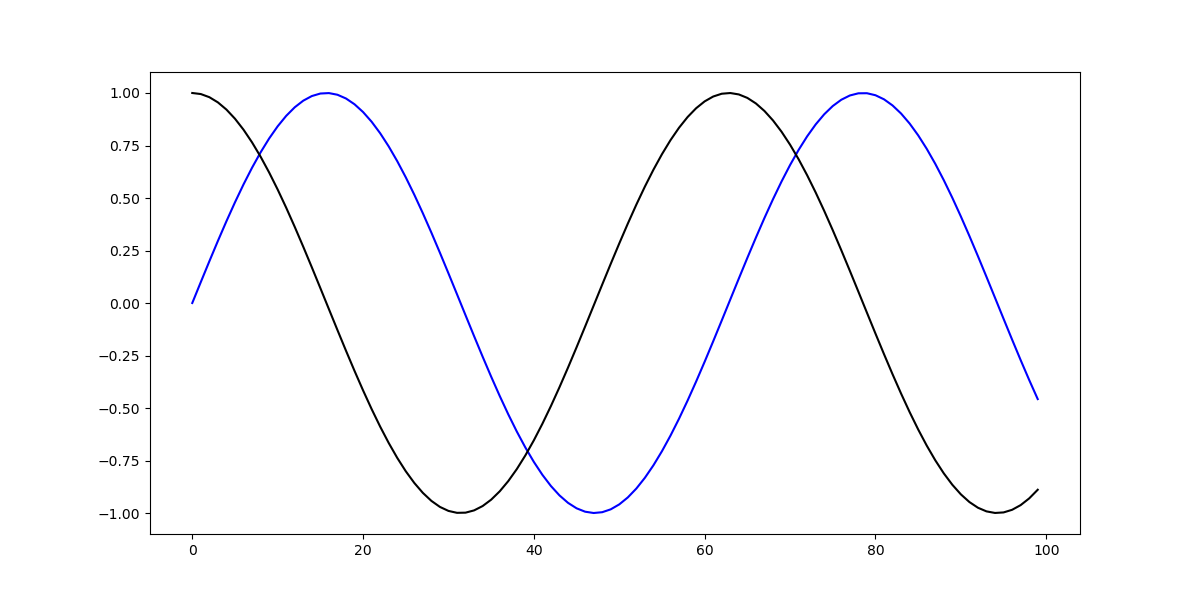Now, we can tweak the range of this axis, which currently goes from 0 to 100.

### Setting Axis Range in Matplotlib

Now, if we'd like to truncate that view, into a smaller one or even a larger one, we can tweak the X and Y limits. These can be accessed either through the PyPlot instance, or the Axes instance.

#### How to Set X-Limit (xlim) in Matplotlib

Let's first set the X-limit, using both the PyPlot and Axes instances. Both of these methods accept a tuple - the left and right limits. So, for example, if we wanted to truncate the view to only show the data in the range of 25-50 on the X-axis, we'd use xlim([25, 50]):

fig, ax = plt.subplots(figsize=(12, 6))

x = np.arange(0, 10, 0.1)
y = np.sin(x)
z = np.cos(x)

ax.plot(y, color='blue', label='Sine wave')
ax.plot(z, color='black', label='Cosine wave')

plt.xlim([25, 50])
plt.show()


This limits the view on the X-axis to the data between 25 and 50 and results in: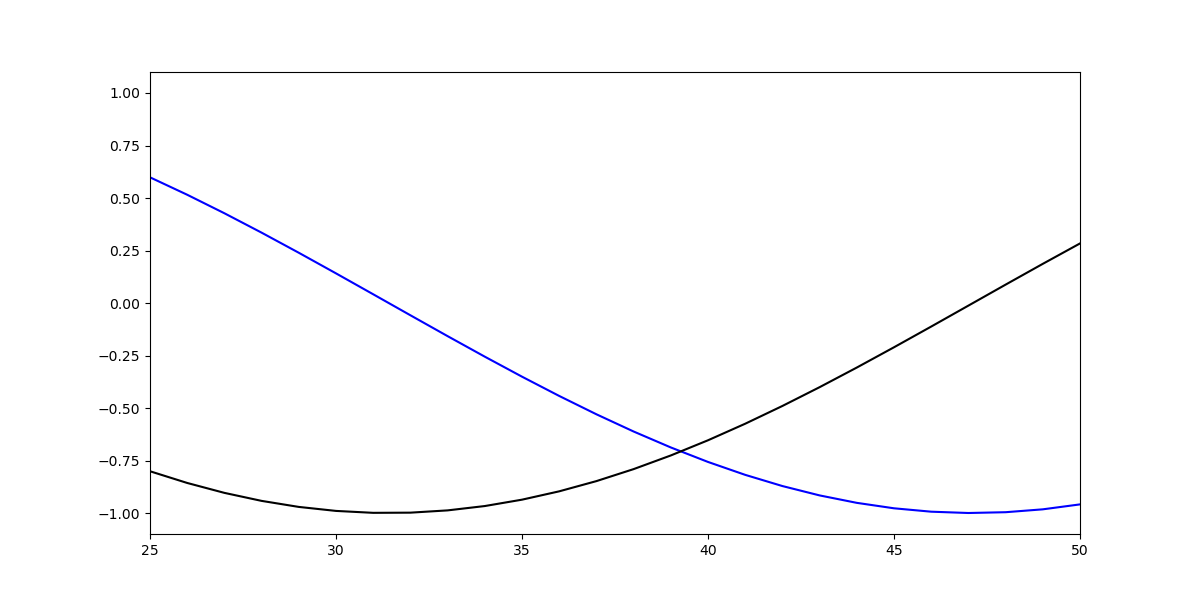This same effect can be achieved by setting these via the ax object. This way, if we have multiple Axes, we can set the limit for them separately:

import matplotlib.pyplot as plt
import numpy as np

fig = plt.figure(figsize=(12, 6))

x = np.arange(0, 10, 0.1)
y = np.sin(x)
z = np.cos(x)

ax = fig.add_subplot(121)
ax2 = fig.add_subplot(122)

ax.set_title('Full view')
ax.plot(y, color='blue', label='Sine wave')
ax.plot(z, color='black', label='Cosine wave')

ax2.set_title('Truncated view')
ax2.plot(y, color='blue', label='Sine wave')
ax2.plot(z, color='black', label='Cosine wave')

ax2.set_xlim([25, 50])

plt.show()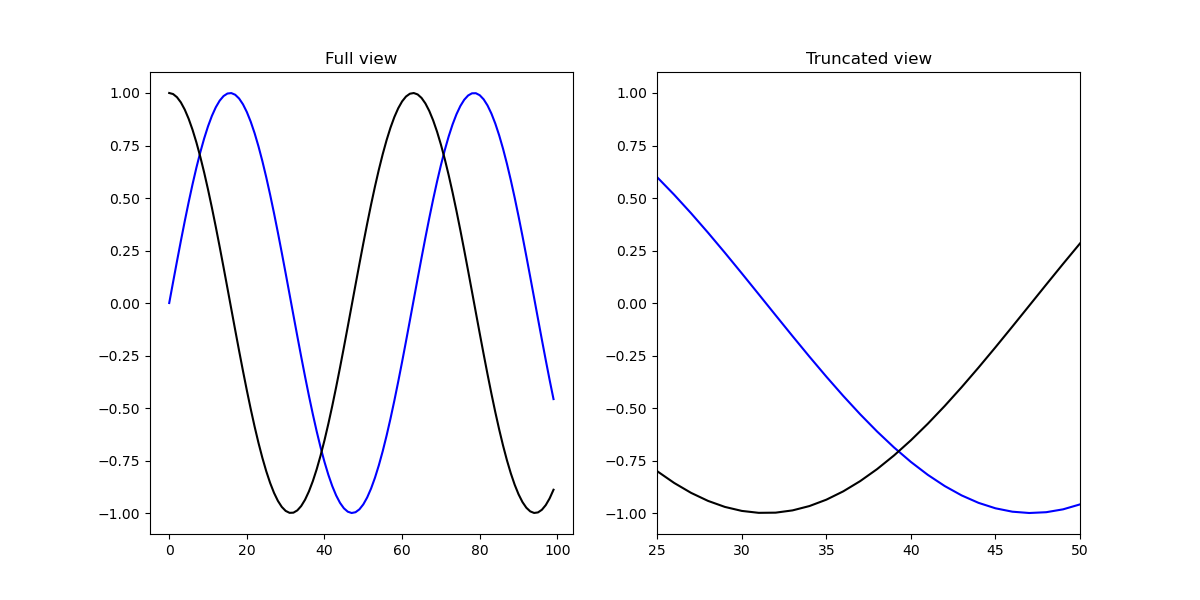#### How to Set Y-Limit (ylim) in Matplotlib

Now, let's set the Y-limit. This can be achieved with the same two approaches:

ax.plot(y, color='blue', label='Sine wave')
ax.plot(z, color='black', label='Cosine wave')

plt.ylim([-1, 0])


Or:

ax.plot(y, color='blue', label='Sine wave')
ax.plot(z, color='black', label='Cosine wave')

ax.set_ylim([-1, 0])


Both of which result in: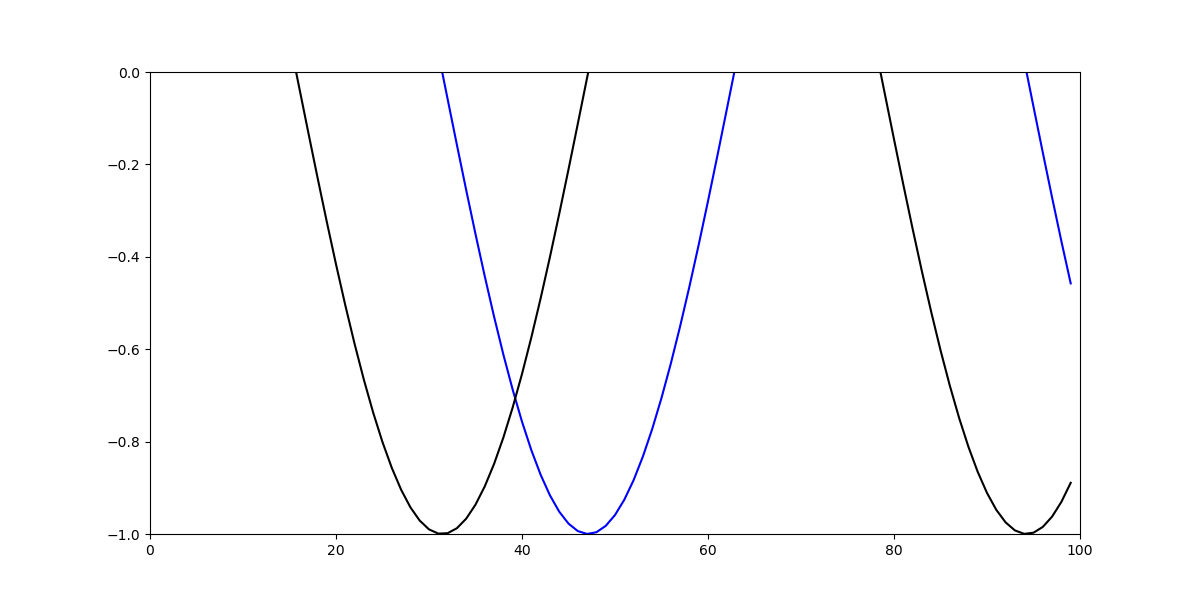### Conclusion

In this tutorial, we've gone over how to set the axis range (i.e. the X and Y limits) using Matplotlib in Python.

If you're interested in Data Visualization and don't know where to start, make sure to check out our bundle of books on Data Visualization in Python:

## Better understand your data with visualizations.

•  30-day no-questions money-back guarantee
•  Beginner to Advanced
•  Updated regularly (latest update June 2021)
•  Updated with bonus resources and guides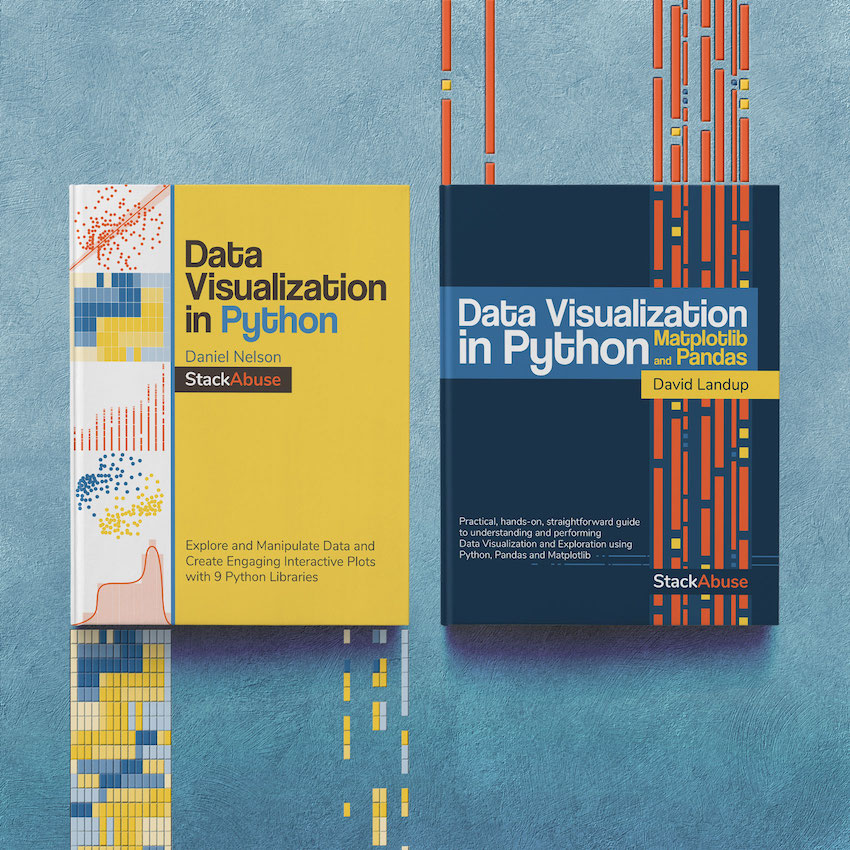Become dangerous with Data Visualization

✅ 30-day no-question money-back guarantee

✅ Beginner to Advanced

✅ Updated regularly for free (latest update in April 2021)

✅ Updated with bonus resources and guides

Data Visualization in Python with Matplotlib and Pandas is a book designed to take absolute beginners to Pandas and Matplotlib, with basic Python knowledge, and allow them to build a strong foundation for advanced work with theses libraries - from simple plots to animated 3D plots with interactive buttons.

It serves as an in-depth, guide that'll teach you everything you need to know about Pandas and Matplotlib, including how to construct plot types that aren't built into the library itself.

Data Visualization in Python, a book for beginner to intermediate Python developers, guides you through simple data manipulation with Pandas, cover core plotting libraries like Matplotlib and Seaborn, and show you how to take advantage of declarative and experimental libraries like Altair. More specifically, over the span of 11 chapters this book covers 9 Python libraries: Pandas, Matplotlib, Seaborn, Bokeh, Altair, Plotly, GGPlot, GeoPandas, and VisPy.

It serves as a unique, practical guide to Data Visualization, in a plethora of tools you might use in your career.

Last Updated: May 5th, 2021

## Improve your dev skills!

Get tutorials, guides, and dev jobs in your inbox.

No spam ever. Unsubscribe at any time. Read our Privacy Policy.

# Prepping for an interview?

• Improve your skills by solving one coding problem every day
• Get the solutions the next morning via email
• Practice on actual problems asked by top companies, like:

© 2013-2021 Stack Abuse. All rights reserved.